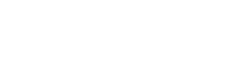# Dividend Payout Ratio

## Dividend Payout Ratio Definition

Dividend Payout Ratio is the amount of dividend that a company gives out to its shareholders out of its current earnings. The earnings that the company retains with it after paying off the dividends is called as “Retained Earnings” and is invested by the company for its growth and future. In other words, the total earnings of a company comprised of two essential parts i.e. Dividend payout ratio (the dividend it pays) and Retention Ratio (the amount it retains for re-investing in the business).

## How to Calculate Dividend Payout Ratio?

The dividend payout can be calculated using two different equations, each of which can be easily computed from the data in your financial statements and give the same results.

### Formula / Equation

It is important to note that both the numerator and denominator in this equation do not include any preference dividends or preference shares and only include the dividend, which is paid to common equity shareholders. To arrive at the numerator, you can divide the total dividend payable to equity shareholders by the total number of outstanding equity shares of the company. Similarly, for calculating the earnings per share, divide the total earnings of the company by the number of equity shares.In this equation too, preference dividend or shares are not to be included in the calculation of total dividend and net income. This equation is relatively simpler as it requires lesser calculations and you can straight away pick up the concerned figures from the financial statements.## Example

Let us understand the concept with a practical example.

Suppose, the annual dividend paid by ABC Company is Rs. 30 per share. The net earnings of ABC Company during this period is Rs. 100 per share.

Using the below formula, we can calculate the ratio as follows:As 30% is the dividend payout ratio, it automatically means that 70% (100-30) is the retention ratio with which the company has decided to fuel its long-term objectives and overall growth.

## Analysis

Now it may seem that a company having a higher dividend payout ratio is at a better position than a company with a lower ratio, however, it is not necessarily true. What needs to be looked at is how consistently the company has been paying out those dividends. A company with a stable 20% payout ratio since say, 10 years, is more trustworthy than a company with a payout ratio of 30% but which is consistently on the decline over the years. In the latter case, it is possible that in the coming years the company may not be able to pay dividends at all. Consistency or long-term trends are what you should focus on, more than the dividend rate.It is also imperative to know that most of the startup companies or the companies that are looking to expand their business, understandably, have a lower dividend payout ratio, as they want to retain as much of the earnings and use it for bringing the company up. These companies start giving higher dividends when they do not have sufficient projects to invest.

Therefore, if an investor is looking for a steady dividend income you can always keep this factor in mind and look for companies that have been in the running for a long time and with a good performance record. Though beware of extremely high dividend payout ratios as that means the company is giving out most of its earnings and relying on a very negligible amount for investing in its future operations, which is not a good sign.

Conclusion

Dividend Payout Ratio lets an investor calculate the percentage of the dividend that the company has decided to distribute out of its net earnings. It is of utmost importance to keep a track of the ratio of a company over the years to take a call as to which company would be the right choice to invest in.1–4

Share Knowledge if you liked